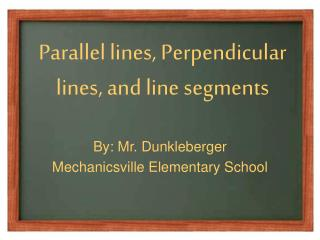DownloadDownload PresentationParallel lines, Perpendicular lines, and line segments

# Parallel lines, Perpendicular lines, and line segments

Télécharger la présentation## Parallel lines, Perpendicular lines, and line segments

- - - - - - - - - - - - - - - - - - - - - - - - - - - E N D - - - - - - - - - - - - - - - - - - - - - - - - - - -
##### Presentation Transcript

1. Parallel lines, Perpendicular lines, and line segments By: Mr. Dunkleberger Mechanicsville Elementary School

2. What is a line? • Line- a straight path in a plane that goes on forever in opposite directions Example: • line AB or line BA

3. What is a point? • point • An exact location in space, usually represented by a dot Example:point A

4. What is a line segment? • line segment • A part of a line that includes two points, called endpoints, and all of the points between them Example: • line segment AB  or line segment BA

5. What is a ray? • ray • A part of a line, with one endpoint, that continues without end in one direction Example:ray CD

6. What is a parallel line? • parallel lines • Lines in a plane that never intersect Example:

7. What is a perpendicular line? • perpendicular lines • Two lines that intersect to form four right anglesExample:

8. Lines & Angles

9. ray

10. . point

11. line segment

12. angle

13. line

14. right angle

15. obtuse angle

16. acute angle

17. parallel lines

18. intersecting lines

19. perpendicular lines

20. What is it? Is the following shape a parallel line, a perpendicular line, or an intersecting line?

21. What is it? Is the following shape a parallel line, a perpendicular line, or an intersecting line?

22. What is it? Is the following shape a parallel line, a perpendicular line, or an intersecting line?

23. What is it? Is the following shape a parallel line, a perpendicular line, or an intersecting line?

24. What is it? Is the following shape a parallel line, a perpendicular line, or an intersecting line?

25. What is it? Is the following shape a parallel line, a perpendicular line, or an intersecting line?

26. What is it? Is the following shape a parallel line, a perpendicular line, or an intersecting line?

27. What is it? Is the following shape a parallel line, a perpendicular line, or an intersecting line?

28. What is it? Is the following a point, a line, a line segment, or a ray?

29. What is it? Is the following a point, a line, a line segment, or a ray?

30. What is it? Is the following a point, a line, a line segment, or a ray?

31. What is it? Is the following a point, a line, a line segment, or a ray?

32. What is it? Is the following a point, a line, a line segment, or a ray?

33. What is it? Is the following a point, a line, a line segment, or a ray?

34. What is it? Is the following a point, a line, a line segment, or a ray?